# 逻辑回归模型的贝叶斯分析

### 贝叶斯推断rng(0,'twister'); n = 20; sigma = 50; x = normrnd(10,sigma,n,1); mu = 30; tau = 20; theta = linspace(-40, 100, 500); y1 = normpdf(mean(x),theta,sigma/sqrt(n)); y2 = normpdf(theta,mu,tau); postMean = tau^2*mean(x)/(tau^2+sigma^2/n) + sigma^2*mu/n/(tau^2+sigma^2/n); postSD = sqrt(tau^2*sigma^2/n/(tau^2+sigma^2/n)); y3 = normpdf(theta, postMean,postSD); plot(theta,y1,'-', theta,y2,'--', theta,y3,'-.') legend('Likelihood','Prior','Posterior') xlabel('\theta')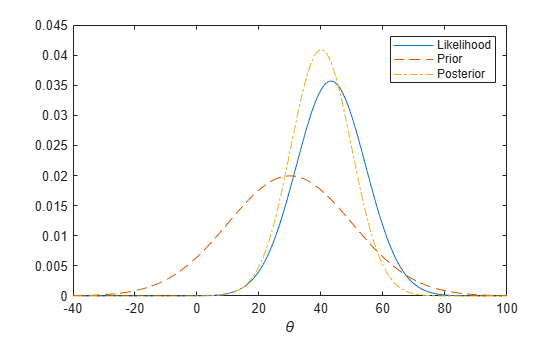### 汽车试验数据

% A set of car weights weight = [2100 2300 2500 2700 2900 3100 3300 3500 3700 3900 4100 4300]'; weight = (weight-2800)/1000; % recenter and rescale % The number of cars tested at each weight total = [48 42 31 34 31 21 23 23 21 16 17 21]'; % The number of cars that have poor mpg performances at each weight poor = [1 2 0 3 8 8 14 17 19 15 17 21]'; 

### 逻辑回归模型logitp = @(b,x) exp(b(1)+b(2).*x)./(1+exp(b(1)+b(2).*x)); 

prior1 = @(b1) normpdf(b1,0,20); % prior for intercept prior2 = @(b2) normpdf(b2,0,20); % prior for slope 

post = @(b) prod(binopdf(poor,total,logitp(b,weight))) ... % likelihood * prior1(b(1)) * prior2(b(2)); % priors 

b1 = linspace(-2.5, -1, 50); b2 = linspace(3, 5.5, 50); simpost = zeros(50,50); for i = 1:length(b1) for j = 1:length(b2) simpost(i,j) = post([b1(i), b2(j)]); end; end; mesh(b2,b1,simpost) xlabel('Slope') ylabel('Intercept') zlabel('Posterior density') view(-110,30)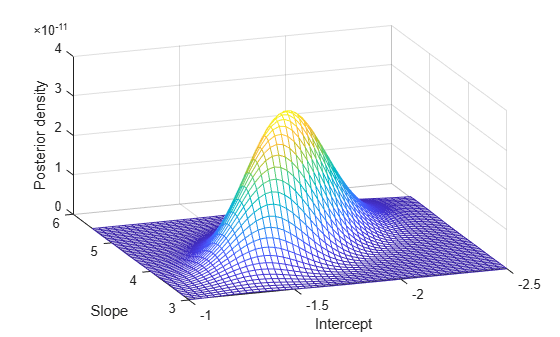### 切片抽样

initial = [1 1]; nsamples = 1000; trace = slicesample(initial,nsamples,'pdf',post,'width',[20 2]); 

### 抽样器输出分析

subplot(2,1,1) plot(trace(:,1)) ylabel('Intercept'); subplot(2,1,2) plot(trace(:,2)) ylabel('Slope'); xlabel('Sample Number');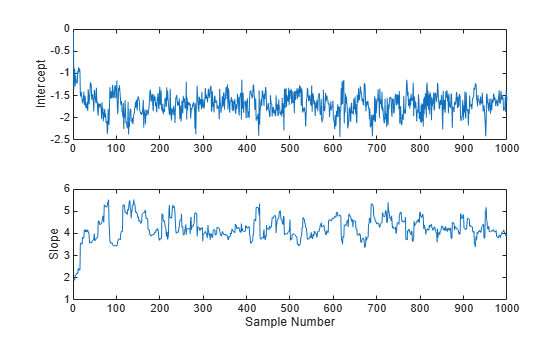movavg = filter( (1/50)*ones(50,1), 1, trace); subplot(2,1,1) plot(movavg(:,1)) xlabel('Number of samples') ylabel('Means of the intercept'); subplot(2,1,2) plot(movavg(:,2)) xlabel('Number of samples') ylabel('Means of the slope');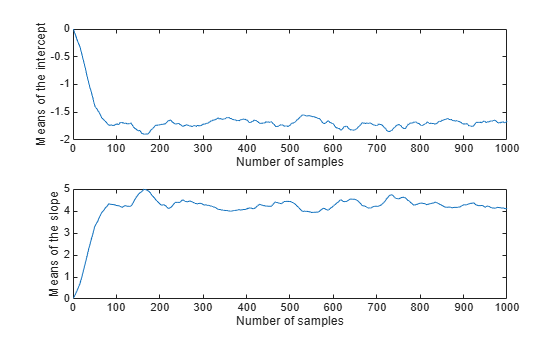trace = slicesample(initial,nsamples,'pdf',post, ... 'width',[20 2],'burnin',50); subplot(2,1,1) plot(trace(:,1)) ylabel('Intercept'); subplot(2,1,2) plot(trace(:,2)) ylabel('Slope');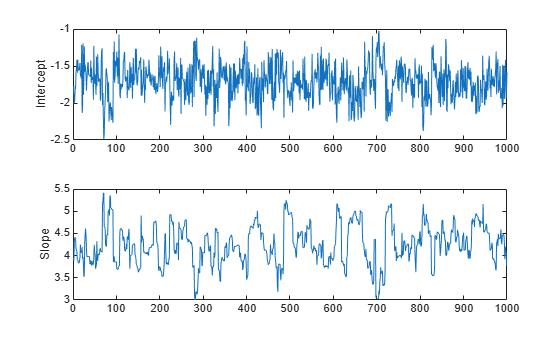trace = slicesample(initial,nsamples,'pdf',post,'width',[20 2], ... 'burnin',50,'thin',10); 

F = fft(detrend(trace,'constant')); F = F .* conj(F); ACF = ifft(F); ACF = ACF(1:21,:); % Retain lags up to 20. ACF = real([ACF(1:21,1) ./ ACF(1,1) ... ACF(1:21,2) ./ ACF(1,2)]); % Normalize. bounds = sqrt(1/nsamples) * [2 ; -2]; % 95% CI for iid normal labs = {'Sample ACF for intercept','Sample ACF for slope' }; for i = 1:2 subplot(2,1,i) lineHandles = stem(0:20, ACF(:,i) , 'filled' , 'r-o'); lineHandles.MarkerSize = 4; grid('on') xlabel('Lag') ylabel(labs{i}) hold on plot([0.5 0.5 ; 20 20] , [bounds([1 1]) bounds([2 2])] , '-b'); plot([0 20] , [0 0] , '-k'); hold off a = axis; axis([a(1:3) 1]); end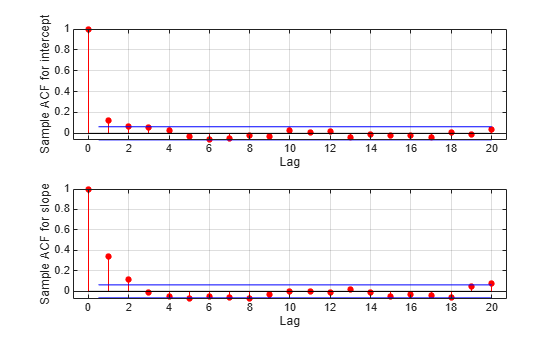### 推断模型参数

subplot(1,1,1) hist3(trace,[25,25]); xlabel('Intercept') ylabel('Slope') zlabel('Posterior density') view(-110,30)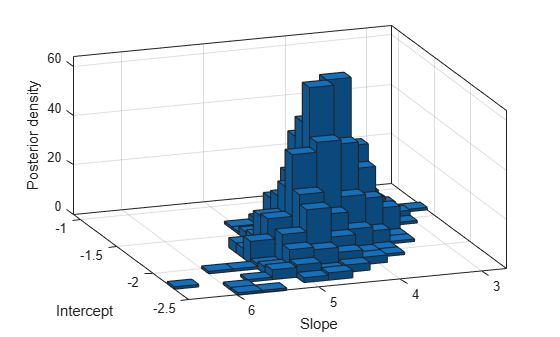subplot(2,1,1) hist(trace(:,1)) xlabel('Intercept'); subplot(2,1,2) ksdensity(trace(:,2)) xlabel('Slope');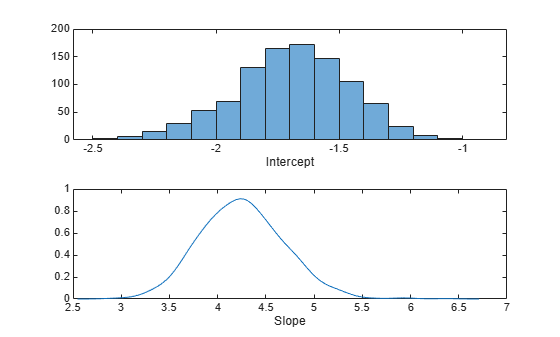csum = cumsum(trace); subplot(2,1,1) plot(csum(:,1)'./(1:nsamples)) xlabel('Number of samples') ylabel('Means of the intercept'); subplot(2,1,2) plot(csum(:,2)'./(1:nsamples)) xlabel('Number of samples') ylabel('Means of the slope');bHat = mean(trace) 
bHat = -1.6931 4.2569 

### 总结

Statistics and Machine Learning Toolbox™ 提供了多种函数，让您能够轻松地指定似然和先验。您也可以将它们结合起来用于推断后验分布。使用 slicesample 函数，您可以通过马尔可夫链蒙特卡罗模拟在 MATLAB 中执行贝叶斯分析。甚至在使用标准随机数生成函数难以抽样的后验分布问题中也可以使用此函数。